# 《模型思维》阅读指引

9个月前 (01-04) 639次浏览

# 《模型思维》阅读指引

## 1.正态分布(Normal Distribution)

### 1.1 函数（Function）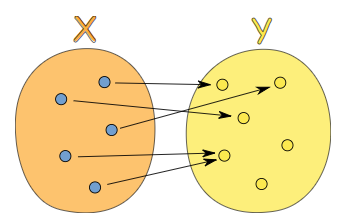1. 每一个元素的意思是X里的所有元素都与Y里的某一个元素有关系，但Y里的一些元素可能和X里的元素没有关系
2. 独一的值的意思是函数是单值的。一个输入值不能有多于一个输出值，所以f(x)=7或9是不允许的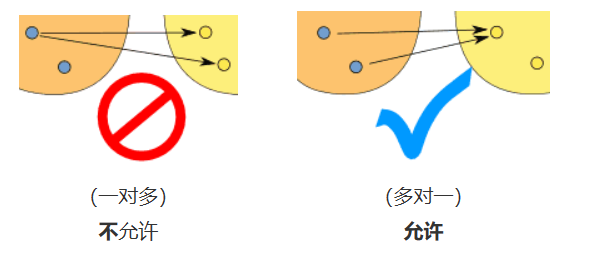### 1.2 随机变量（Random variable）

X={正面，反面}

X={0，1}

1. 随机变量是随机实验结果的可能数值的集合
2. 可能数值的集合是它的样本空间
3. 随机变量使用大写字母来表示，例如X或Y
4. 随机变量可以是离散或连续的数据

### 1.3 离散和连续（Discrete Data and Continuous Date）

1.离散数据只能是某些既定的值

• ​ 班上学生的人数（不能有半个学生）
• ​ 掷骰子的结果（2，3，4，5，6，7，8，9，10，11，12）

2.连续数据可以是一个范围里任何的值

• ​ 身高：可以是任何的值（人类身高范围里）
• ​ 体重：狗的体重
• ​ 长度：叶子的长度
• ​ ……

### 1.4 试验和样本空间（Experiment and Sample Space）

• 梅花5是个样本点
• 红心国王是个样本点
• 国王不是样本点，因为有4个国王

• 抛硬币得到反面是个事件
• 掷骰子拿到5是个事件
• 从一副扑克牌选一张国王是个事件
• 掷骰子得到偶数（2，4，6）也是个事件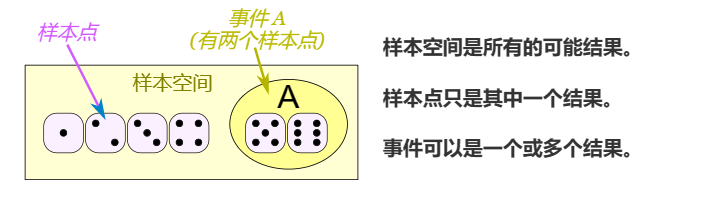### 1.5 平均（ Mean）

#### 1.5.1 算术平均数（ Arithmetic Mean）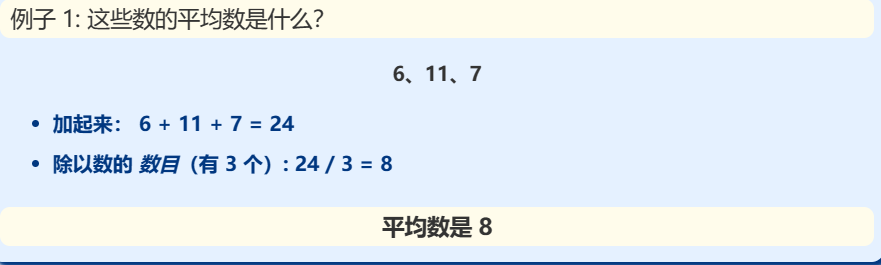#### 1.5.2 几何平均数（ Geometric Mean）

\sqrt[n]{a_1\times a_2\times a_3\times ….\times a_n}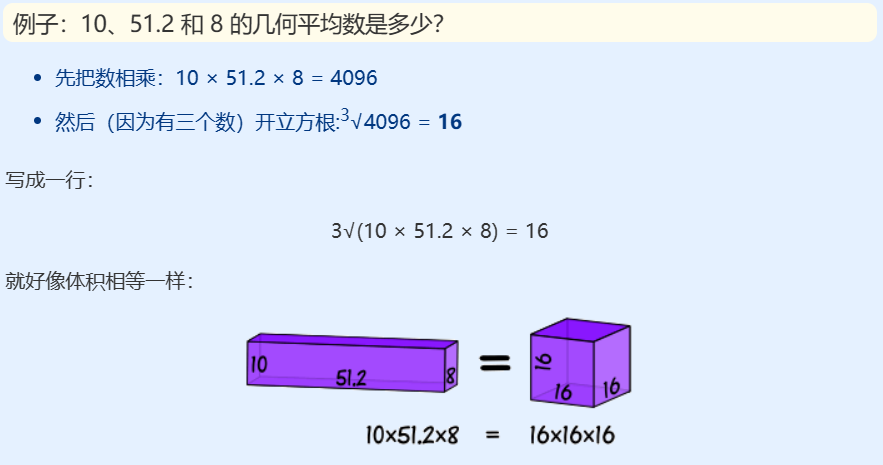#### 1.5.3 加权平均数（Weighted Mean）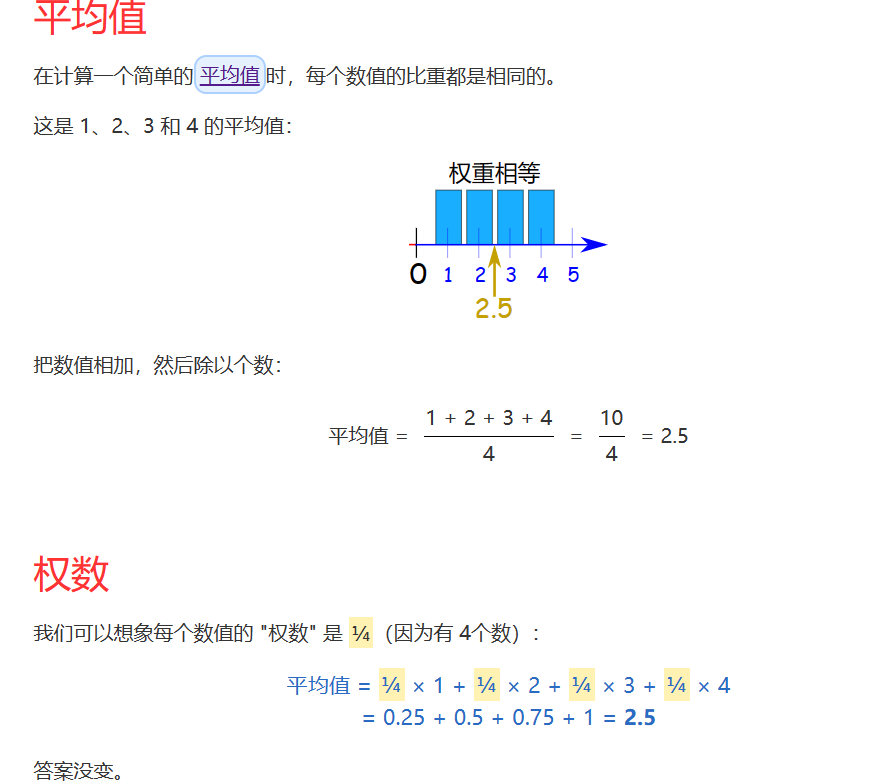### 1.6 方差和标准差（Standard Deviation and Variance）

#### 1.6.1 方差

1. 求数值的平均
2. 从每一个数值减去平均，然后求差的平方
3. 求结果的平均

1.第一步求平均：

2.第二步每个数值减去平均，然后求差的平方

|600-394|^2,|470-394|^2,|170-394|^2,|430-394|^2,|300-394|^2

3.求结果的平均

#### 1.6.2 标准差

\sigma = \sqrt{21704} = 147.32 =147

### 1.7 期望（Expected Value）

\mu =\sum\limits_{i=1}\limits^n{} x_i·p_i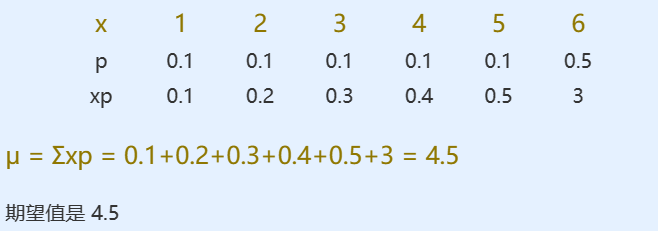#### 1.7.1 离散随机分布的方差

Var(X) =\sum\limits_{i=1}\limits^n p_i·(x_i-\mu)^2 = \sum\limits_{i=1}\limits^n(p_i·x_i^2)-\mu^2

\mu =\sum\limits_{i=1}\limits^n{} x_i·p_i

#### 1.7.2 等概率值的方差

1.6.1小节中，默认每个数值对应的概率都是相等的，它的方差通用公式为

Var(X) = \sigma^2=\frac{1}{n}\sum\limits_{i=1}\limits^n(x_i-\mu)^2